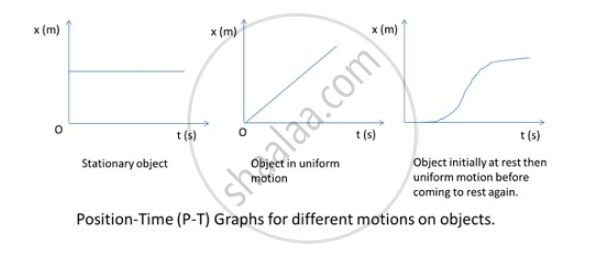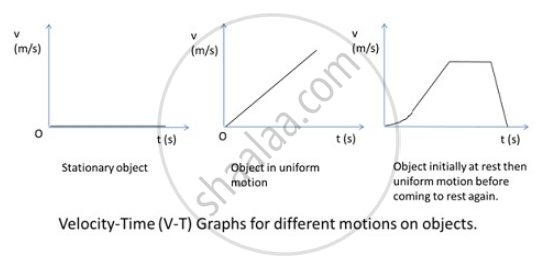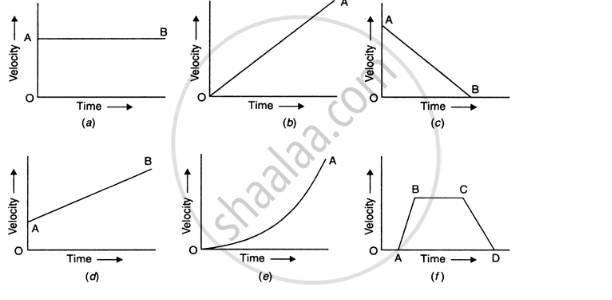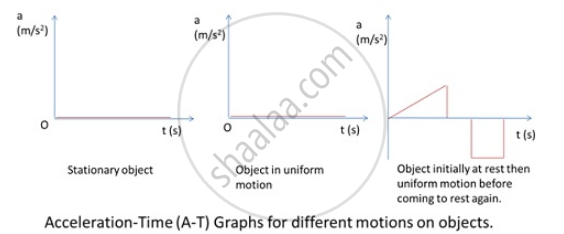# Position-time, Velocity-time and Acceleration-time Graphs

## The Position-Time GraphThe position-time graph describes the motion of an object over a period of time. Time in seconds is conventionally plotted on the x-axis and the position of the object in meters is plotted along the y-axis. The slope of the position-time graph reveals important information about the velocity of the object.

Slope of the Position-Time Graph
The slope of a position-time graph reveals the type of velocity an object undergoes during its motion. A constant slope of a position-time graph indicates a constant velocity. A varying slope of a position-time graph indicates a changing velocity. The direction of the slope of the position-time graph indicates the sign of the velocity. For example, if it slopes downward, from left to right, the velocity is negative.

The Velocity-Time GraphThe velocity-time graph of an object reveals the speed at which an object is moving at a given time and whether it is slowing down or speeding up. Time in seconds is usually plotted on the x-axis while the velocity in meters per second is usually plotted along the y-axis. Objects moving at a constant rate have a straight-line velocity-time graph. Objects moving at variable speeds have sloping, linear velocity graphs.

Slope of the Velocity-Time Graph
The slope of the velocity-time graph reveals the acceleration of an object. If the slope of the velocity-time graph is a horizontal line, the acceleration is 0. This means the object is either at rest or moving at a constant speed, without speeding up or slowing down. If the slope is positive, the acceleration is increasing. If slope is negative, acceleration is decreasing.(a) Since the velocity-time graph is a straight line, the body has uniform velocity.
(b)The body has uniform acceleration and its initial velocity is zero because the line starts from the origin.
(c)The body has some initial velocity and is under uniform retardation.
(d)The body has some initial velocity and uniform acceleration.
(e)The body has zero initial velocity and it has variable acceleration.
(f)The body is at rest from O to A, it has uniform acceleration from A to B, it has uniform velocity from B to C and from C to D, the body is under uniform retardation.

### The Acceleration-Time GraphThe vertical axis represents the acceleration of the object.

Slope of the Acceleration-Time Graph
The slope of an acceleration graph represents a quantity called the jerk. The jerk is the rate of change of the acceleration.
The slope of an acceleration graph represents a quantity called the jerk. The jerk is the rate of change of the acceleration.
For an acceleration graph, the slope can be found from
slope=(rise)/(run)=(a_2-a_1)/(t_2-t_1)=(Δa)/(Δt),
as can be seen in the diagram below. This slope, which represents the rate of change of acceleration, is defined to be the jerk.
jerk=(Δa)/(Δt)
The area under an acceleration graph represents the change in velocity. In other words, the area under the acceleration graph for a certain time interval is equal to the change in velocity during that time interval.
area=Δv

If you would like to contribute notes or other learning material, please submit them using the button below.

### Shaalaa.com

Straight line motion Part 4 (Distance time graph) [00:07:35]
S
0%